# Volume of a Rectangular Prism made of Unit Cubes Online Quiz

Following quiz provides Multiple Choice Questions (MCQs) related to Volume of a Rectangular Prism made of Unit Cubes. You will have to read all the given answers and click over the correct answer. If you are not sure about the answer then you can check the answer using Show Answer button. You can use Next Quiz button to check new set of questions in the quiz.Q 1 - Find the length, width and height of the following rectangular prism. Then find its volume.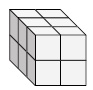### Explanation

Step 1:

The given prism has length = 2; width = 2; height = 3

Step 2:

The volume of prism V = l × w × h = 2 × 2 × 3

= 12 cubic units.

Q 2 - Find the length, width and height of the following rectangular prism. Then find its volume.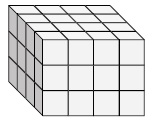### Explanation

Step 1:

The given prism has length = 4; width = 3; height = 4

Step 2:

The volume of prism V = l × w × h = 4 × 3 × 4

= 48 cubic units.

Q 3 - Find the length, width and height of the following rectangular prism. Then find its volume.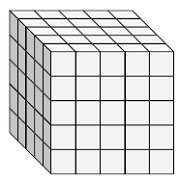### Explanation

Step 1:

The given prism has length = 5; width = 5; height = 5.

Step 2:

The volume of prism V = l × w × h = 5 × 5 × 5

= 125 cubic units.

Q 4 - Find the length, width and height of the following rectangular prism. Then find its volume.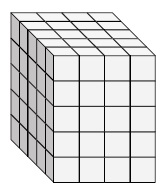### Explanation

Step 1:

The given prism has length = 4; width = 5; height = 5.

Step 2:

The volume of prism V = l × w × h = 4 × 5 × 5

= 100 cubic units.

Q 5 - Find the length, width and height of the following rectangular prism. Then find its volume.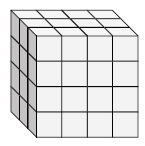### Explanation

Step 1:

The given prism has length = 4; width = 4; height = 3

Step 2:

The volume of prism V = l × w × h = 4 × 4 × 3

= 48 cubic units.

Q 6 - Find the length, width and height of the following rectangular prism. Then find its volume.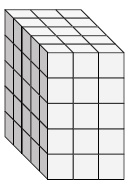### Explanation

Step 1:

The given prism has length = 3; width = 5; height = 5

Step 2:

The volume of prism V = l × w × h = 3 × 5 × 5

= 75 cubic units.

Q 7 - Find the length, width and height of the following rectangular prism. Then find its volume.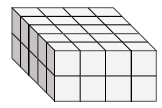### Explanation

Step 1:

The given prism has length = 4; width = 2; height = 5

Step 2:

The volume of prism V = l × w × h = 4 × 2 × 5

= 40 cubic units.

Q 8 - Find the length, width and height of the following rectangular prism. Then find its volume.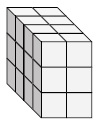### Explanation

Step 1:

The given prism has length = 2; width = 3; height = 4.

Step 2:

The volume of prism V = l × w × h = 2 × 3 × 4

= 24 cubic units.

Q 9 - Find the length, width and height of the following rectangular prism. Then find its volume.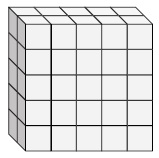### Explanation

Step 1:

The given prism has length = 5; width = 5; height = 2.

Step 2:

The volume of prism V = l × w × h = 5 × 5 × 2

= 50 cubic units.

Q 10 - Find the length, width and height of the following rectangular prism. Then find its volume.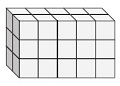### Explanation

Step 1:

The given prism has length = 5; width = 3; height = 2

Step 2:

The volume of prism V = l × w × h = 5 × 3 × 2

= 30 cubic units.